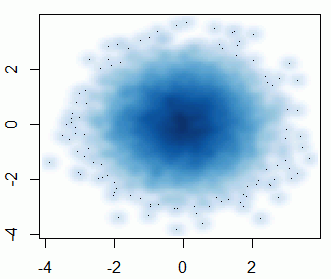# ML Wiki

## Scatter Plot

This is a Plot that can be useful for initial analysis of the data

• it uses Cartesian coordinates to visualize relationships between variables
• So, useful for Bivariate Analysis
plot(pData$JWMNP, pData$WAGP, pch=19, col="blue")
•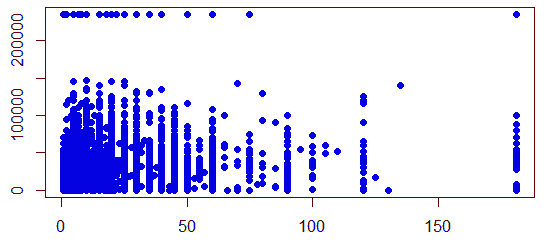## Tips and Tricks

### More Variables

What if we want to include one more variable?

• use color coding
• e.g. can encode sex in color
plot(pData$JWMNP, pData$WAGP, pch=19, col=pData\$SEX, cex=0.5)
•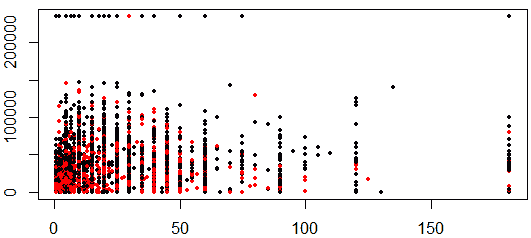### Lots of Data

How to visualize when there are many data points in your data?

x = rnorm(10000)
y = rnorm(10000)
plot(x, y, pch=19)

• not very visible
•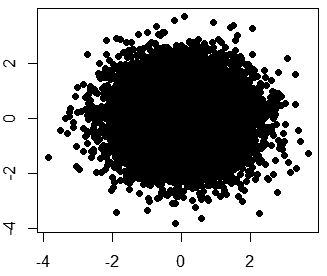#### Sampling

Sample you data

• and plot just the part
sampledValues = sample(1:10000, size=1000, replace=F)
plot(x[sampledValues], y[sampledValues], pch=19)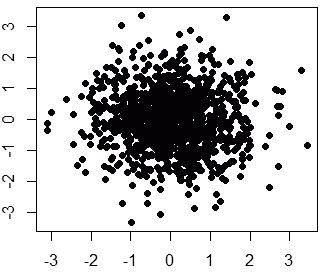#### Showing Density

• how to show the density of some area?
• smoothScatter(x, y)
•